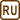## Wheel Rotation

A wheel is rotating with frequency of 25 Hz. By braking we can achieve that the rotation will be linearly decelerating and the wheel will stop after 30 s from the beginning of the braking. Evaluate the angular acceleration and the number of turns the wheel makes from the beginning of the braking till stopping.

• #### Given values

 f = 25 Hz wheel rotation frequency t0 = 30 s time after which the wheel stops ε = ? (s-2) wheel angular acceleration N = ? number of turns the wheel makes from the beginning of braking till stopping
• #### Hint 1 – Angular acceleration of the wheel

What is the relation between the instantaneous value of angular velocity and angular acceleration of the wheel?

What was the initial angular velocity the wheel had before it started decelerating? What was the angular speed at time t0 ?

• #### Hint 2 – Number of revolutions - numerical solution

How do you find out how many turns the wheel has made in a given time?

Use the angle which the position vector sweeps out around the center of the wheel in the given time. Express it using the magnitude of angular acceleration that you know from the previous hint and the angular speed before braking.

• #### Hint 3 – Number of turns - graphical solution

Number of revolutions can be found graphically as well.

Draw a graph of the rotating wheel frequency dependence on time. Where in the graph is the number of turns hidden?

• #### Complete Solution

There is a formula for the instantaneous angular speed:

$\omega\,=\,\omega_0+\varepsilon t\,,$ $\omega_0\,=\,2\pi f\,=\,50\pi\,\mathrm{s^{-1}}\,.$

At time t = t0 there will be ω = 0, so:

$\omega_0+\varepsilon{t_0}\,=\,0\,,$ $\varepsilon\,=\,-\frac{\omega_0}{t_0}\,=\,-\frac{2{\pi}f}{t_0} \,=\, -\frac{50}{30}\pi\,\mathrm{s^{-2}}\,=\,-5.24\,\mathrm{s^{-2}}\,.$

Position vector sweeps out with respect to the centre in time t0 an angle:

$\psi_0\,=\,\omega_0t_0+\frac{\varepsilon{t_0^{2}}}{2}\,=\, 2{\pi}ft_0 + \frac{\varepsilon{t_0^{2}}}{2}\,,$ $\psi_0\,=\, 1500\pi-\frac{5}{6}\pi900\,=\,750\pi\,.$

Number of revolutions in time t0:

$N\,=\,\frac{\psi_0}{2\pi}\,=\,\frac{750\pi}{2\pi}\,=\,375\,.$

Graphical solution:

Number of turns corresponds to the area under the curve.

Angular acceleration of the wheel ε is:

$\varepsilon\,=\,-\frac{2{\pi}f}{t_0} \,=\, -5.24\,\mathrm{s^{-2}}\,.$

Number of turns N that the wheel makes from the beginning of braking is:

$N\,=\,\frac{\psi_0}{2\pi}\,=\,ft_0 + \frac{\varepsilon{t_0^{2}}}{4\pi}\,=\,375\,.$
• #### Number of turns – one more approach

Number of revolutions the wheel makes can be found using the following method as well. Frequency changes with time according to equation:

$f\left(t\right)\,=\, f_0 - kt\,,$

where

$k\,=\,\frac{25}{30}\,.$

(slope of the line from graph 3 in Hint 3)

Number of turns the wheel makes from the beginning of braking till stopping can be calculated as an integral of function f(t) in the particular time interval (area under the curve):

$N\,=\,\int_{0}^{30}{f(t)}\mathrm{d}t\,=\, \int_{0}^{30}{(f_0 - \frac{25}{30}t)}\mathrm{d}t\,,$ $N\,=\,\int_{0}^{30}{(f_0 - \frac{5}{6}t)}\mathrm{d}t\,=\, \left[f_0t - \frac{5t^{2}}{6{\cdot}2}\right]_0^{30}\,,$ $N\,=\, 25{\cdot}30 - \frac{5{\cdot}30^{2}}{2{\cdot}6} \,=\, 375\,.$×Original source: Mičkal, K.: Sbírka úloh z technické mechaniky, SNTL, Praha 1988
Zpracováno v diplomové práci Jany Moltašové (2011).